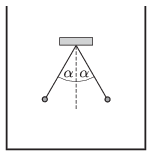Mathematical and Physical Journal
for High Schools
Issued by the MATFUND Foundation
 Already signed up? New to KöMaL?

#Problem P. 4743. (May 2015)

P. 4743. Two insulating threads, which have the same lengths, are suspended at the same point and at their lower end of each, there is a small ebony ball attached. The balls are given the same amount of like charges. The angle between the threads is $\displaystyle 2\alpha=60^\circ$ when the pendulums are inside a container at rest in air. Then the container is filled with petroleum, such that both balls are in the petroleum, far from the walls of the container and from the surface of the liquid. What is the angle between the threads now?Data: The density of ebony is $\displaystyle 1200~{\rm kg}/{\rm m^3}$, the density of petroleum is $\displaystyle 820~{\rm kg}/{\rm m^3}$. The relative dielectric constant of petroleum is $\displaystyle \varepsilon_{\rm r}=2$.

(5 pont)

Deadline expired on June 10, 2015.

### Statistics:

 43 students sent a solution. 5 points: Asztalos Bogdán, Balogh Menyhért, Bartók Imre, Bekes Nándor, Berta Dénes, Blum Balázs, Boldizsár Bálint, Csathó Botond, Csire Roland, Csorba Benjámin, Fehér Balázs, Forrai Botond, Fülöp Erik, Holczer András, Jakus Balázs István, Kaposvári Péter, Kasza Bence, Kormányos Hanna Rebeka, Kovács Péter Tamás, Körtefái Dóra, Marosvári Kristóf, Németh Flóra Boróka, Olosz Balázs, Öreg Botond, Páhoki Tamás, Pázmán Előd, Sal Kristóf, Szántó Benedek, Szentivánszki Soma , Zöllner András. 4 points: Bugár 123 Dávid, Büki Máté, Fekete Panna, Iván Balázs, Molnár 128 Szilárd, Radnai Bálint, Trócsányi Péter, Varga-Umbrich Eszter. 3 points: 1 student. 2 points: 3 students. 1 point: 1 student.

Problems in Physics of KöMaL, May 2015